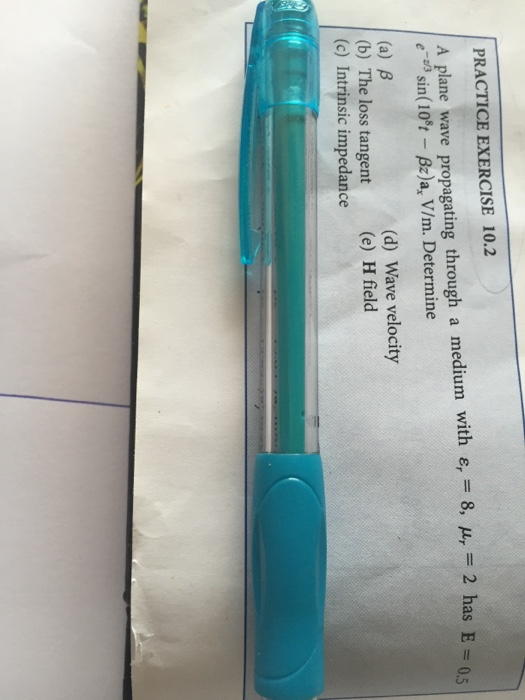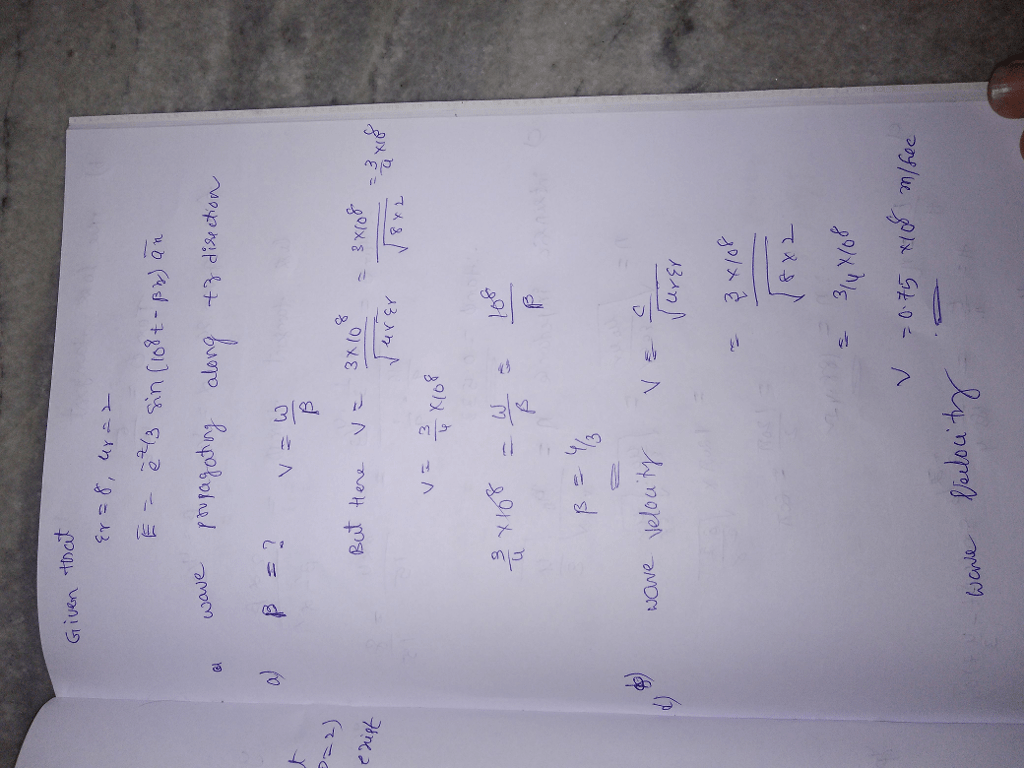# A plane wave propagating through a medium with epsilon_{r} = 8 , mu_{r} = 2 has E = 5 e^ – vec 7 3 sin (10 ^ 8 * t – beta*z) * a_{x} V/m. Determine (a) β (b) The loss tangent (c) Intrinsic impedance (d) Wave velocity (e) H field

Question-AnswerCategory: Electronics EngineeringA plane wave propagating through a medium with epsilon_{r} = 8 , mu_{r} = 2 has E = 5 e^ – vec 7 3 sin (10 ^ 8 * t – beta*z) * a_{x} V/m. Determine (a) β (b) The loss tangent (c) Intrinsic impedance (d) Wave velocity (e) H field

A plane wave propagating through a medium with epsilon_{r} = 8 , mu_{r} = 2 has E = 5 e^ – vec 7 3 sin (10 ^ 8 * t – beta*z) * a_{x} V/m.
Determine (a) β (b) The loss tangent (c) Intrinsic impedance (d) Wave velocity (e) H field